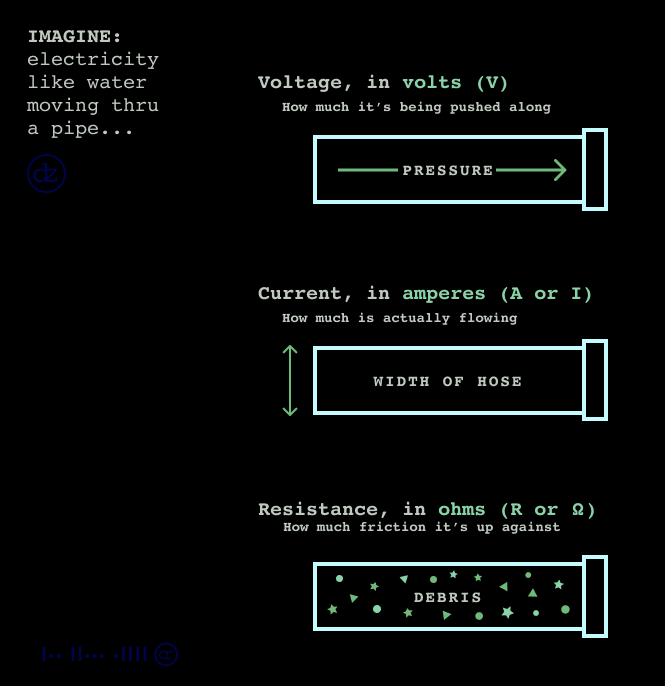<< index

by disinfoniacs #69 & #1

## electrical basics

>>>>

### tldr

• There are six primary electrical concepts: voltage, current, resistance, power, capacitance, and inductance.
• Voltage causes electron flow, measured in volts.
• Current is the movement of electrical charges, measured in amperes.
• Resistance opposes current flow, measured in ohms.
• Power is the rate at which electrical energy is used, measured in watts.
• Capacitance stores energy in electric fields, measured in farads, enabled by capacitors.
• Inductance stores energy in magnetic fields, measured in henries, enabled by inductors.
• Capacitance and inductance are tools to create radio waves.

## in depth

To truly understand radio and be successful in the hobby, you need an understanding of electricity and the tools we use to manipulate it.

Voltage is the electromotive force that initiates the flow of electrons. It is measured in volts, and it is akin to the pressure that pushes water through a pipe. The greater the voltage, the greater the pressure pushing the electrons through the circuit.

The flow of electrons is the current, and it is measured in amperes. Current is the movement of electrical charges, similar to the movement of water through a pipe. The amount of current that flows through a circuit depends on the voltage of the source and the resistance of the circuit.

Resistance is the property that opposes the flow of current and is measured in ohms. Resistance impedes the flow of direct current, alternating current, and RF current, similar to how obstructions in a pipe impede the flow of water. Resistance is influenced by factors such as the material of the wire and the length and thickness of the wire.The next concept you need to grasp is power, which is the rate at which electrical energy is used and is measured in watts. Power is the product of voltage and current, so a circuit with a high voltage and high current will have a high power output. An easy trick to remember the connection is if you use a lot of power, you'll run out of energy.

Capacitance is the ability to store energy in an electric field and is measured in farads. Capacitors are the components that allow us to store energy in electrical fields. A capacitor consists of two conductive plates separated by a dielectric material. When a voltage is applied across the plates, an electric field is established between them, and energy is stored in the field.

Inductance is the ability to store energy in a magnetic field, and it is measured in henries. Inductors are the components that enable us to store energy in magnetic fields. An inductor consists of a coil of wire, and when current flows through the coil, a magnetic field is established around it, and energy is stored in the field. These two concepts are the tools that allow us to create radio waves.

---

<< index